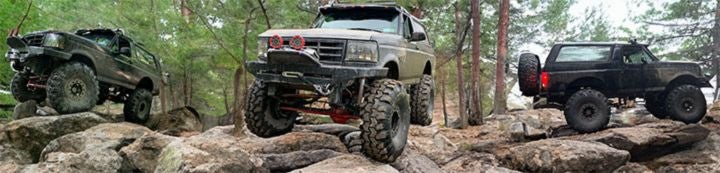1 - 6 of 6 Posts

#### 78Bronco4x4

·
##### Full to Empty 6.5 Seconds
Joined
·
2,579 Posts
Discussion Starter · ·
How much hp and torque does a stock 351m have including tranny gearing, transfer case gearing and diff. gearing.

#### SquattyD

·
Joined
·
3,787 Posts
what trans gear, tcase gear, and rear gears do you have?

tq x trans gear (usually first) x tcasegear (usually low) x rear gear = final tq

so

277 x 2.46 x 1.96 x 3.55 = 4741

and a *massive* crawl ratio of

2.46 x 1.96 x 3.55 = 17 to 1

i think all those numbers are right for a stocker, i did it from memory, but it should be close.

*note* torque in a auto tranny in first gear is multiplied about 2x, just the way the trans works.

#### 78Bronco4x4

·
##### Full to Empty 6.5 Seconds
Joined
·
2,579 Posts
Discussion Starter · ·
Is that in 2 wheel dig or 4 wheel dig? If it was 4wd the power would be multiplied 4x wouldnt it?

#### SquattyD

·
Joined
·
3,787 Posts
no, actually, in 4wd you get less power to each wheel, because the same amount of power produced by then engine is divided 4 ways. It depends on what traction devices you have as well (lockers, etc). But then again, this is all theoretical, because there will be some loss of power due to slipping and such.

At least thats how it seems it works....Im prob wrong.

#### 78Bronco4x4

·
##### Full to Empty 6.5 Seconds
Joined
·
2,579 Posts
Discussion Starter · ·
I think its the other way around IMO. If you have 3.50 gears in both diffs. and shift into 4wd you would have a total ratio of 7.00, 7.46 if you had 3.73:1 ratio (just in the differentials) I figured it this way..

7.00
x 2.46
x 1.96
= 33.75

then

277
x 33.75
= 9348.75

9348.75
x .16
= 2991.6

2991.6
divided by 4
= 747.9 Per Wheel

#### 78Bronco4x4

·
##### Full to Empty 6.5 Seconds
Joined
·
2,579 Posts
Discussion Starter · ·
Damn I wast off. I had to use both diffs. instead of just 1.

3.50
x 2.46
x 1.96
= 7.92

277
x 7.92
= 2193.84

2193.84
- 351.01
= 1842.83

1842.83
divided by 4
= 460.70 Per Wheel

1 - 6 of 6 Posts